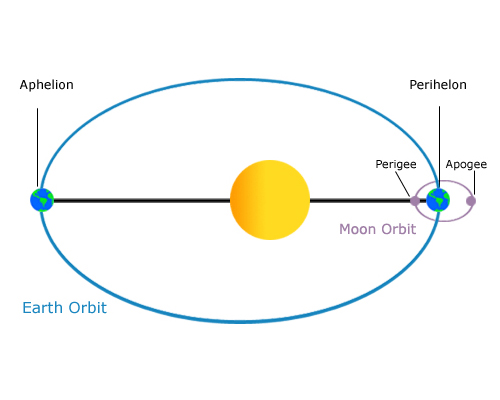Orbital period is defined as the amount of time an object travels around another object. This is in respect to the sidereal period, which is the time required for a celestial body to orbit. In the succeeding paragraph, you will find the varying orbital periods and their respective explanations.

The sidereal period is regarded as an object’s orbital period.

The synodic period is the required time for an object to resurface simultaneously in respect to two other objects. An example would be the Sun, Moon and Earth’s linear relationship. This is the same period that occurs amid two conjunctions where the line of the Earth and the Sun is within similar linear orders.

The draconitic period is the period that passes between two entries where an object crosses two sides of the hemisphere, or what is also known as the ascending node. This is also plane coincidence.

The anomalistic period happens between two entries of an object at its closest point to the attracting body. This is like the planets in relation to the Sun in the Solar System. In space travel terms, an orbital period is the time it takes for a satellite to complete an orbit about its primary.